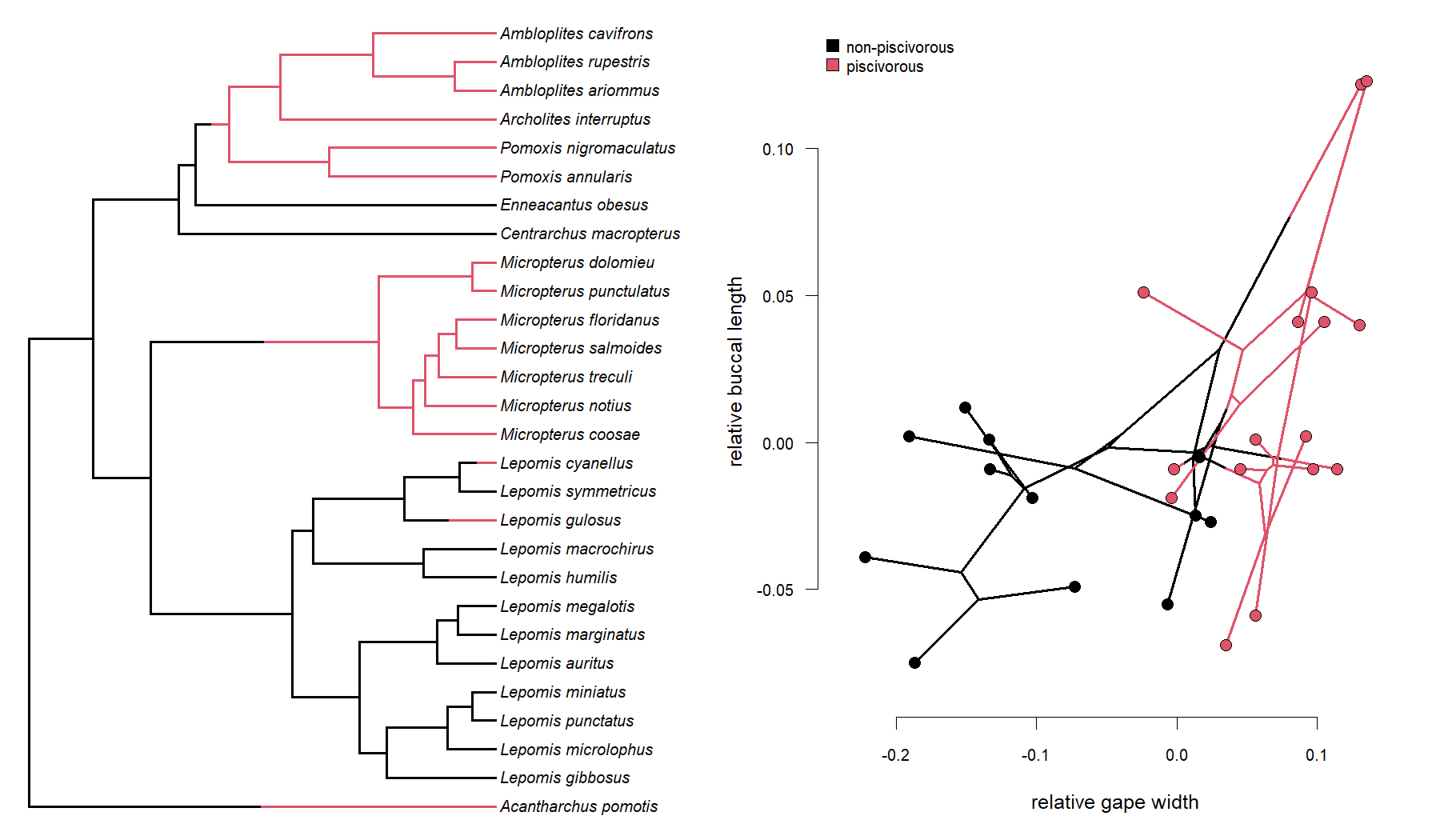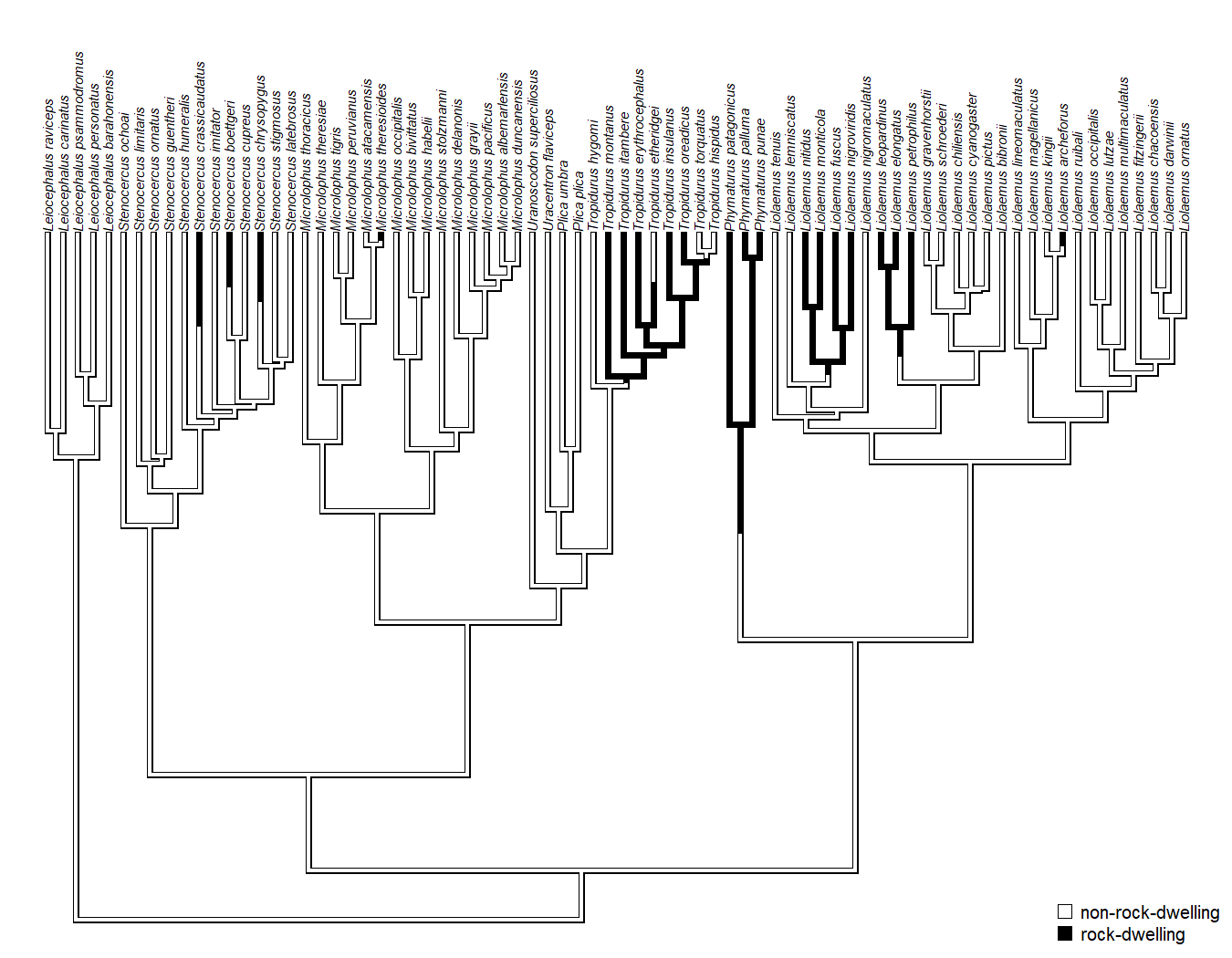## Monday, August 22, 2022

### Improved optimization in `evolvcv.lite` for fitted nested models for heterogeneous evolutionary correlation between traits

In response to some optimization issues a phytools user reported encountered for the function `evolvcv.lite`, I just pushed a handful of changes, which should both improve optimization, and help users to identify certain types of optimization failures.

``````library(phytools)
packageVersion("phytools")
``````
``````##  '1.1.12'
``````

As a reminder, `evolvcv.lite` fits a set of nested heterogeneous correlated Brownian evolution models for two quantitative traits, as described in this recent paper by Ken Toyama, Luke Mahler, and myself.

Here's a quick illustrative demo of how the function works.

In this case, I'm going to load a tree of sunfishes, with feeding mode (piscivory or non-piscivory) mapped onto the edges of the phylogeny. Then I'm going to fit a set of models in which the rate and evolutionary correlation (and both) between two aspects of the buccal morphology are free to vary across the tree according to feeding mode.

Our hypothesis is that piscivory constrains buccal evolution, so there should be a higher evolutionary correlation in piscivorous lineages.

``````## load tree
data(sunfish.tree)
data(sunfish.data)
## create plot showing data
par(mfrow=c(1,2))
plot(sunfish.tree,ftype="i",fsize=0.8)
``````
``````## no colors provided. using the following legend:
##       non      pisc
##   "black" "#DF536B"
``````
``````par(mar=c(5.1,4.1,1.1,1.1),cex.axis=0.8,
las=1)
phylomorphospace(sunfish.tree,
sunfish.data[,2:3],ftype="off",
bty="n",xlab="relative gape width",
ylab="relative buccal length",
node.size=c(0,1.3),node.by.map=TRUE)
legend("topleft",c("non-piscivorous","piscivorous"),
pt.bg=palette()[1:2],pch=22,pt.cex=1.5,
cex=0.8,bty="n")
``````Next, let's run and print our set of models.

``````## fit models
sunfish.fit<-evolvcv.lite(sunfish.tree,sunfish.data[,2:3],models="all models")
``````
``````## Fitting model 1: common rates, common correlation...
## Best log(L) from model 1: 72.1893.
## Fitting model 2: different rates, common correlation...
## Best log(L) from model 2: 77.9869.
## Fitting model 2b: different rates (trait 1), common correlation...
## Best log(L) from model 2b: 75.9661.
## Fitting model 2c: different rates (trait 2), common correlation...
## Best log(L) from model 2c: 75.3309.
## Fitting model 3: common rates, different correlation...
## Best log(L) from model 3: 73.6144.
## Fitting model 3b: different rates (trait 1), different correlation...
## Best log(L) from model 3b: 76.4875.
## Fitting model 3c: different rates (trait 2), different correlation...
## Best log(L) from model 3c: 80.7484.
## Fitting model 4: no common structure...
## Best log(L) from model 4: 81.2393.
``````
``````print(sunfish.fit)
``````
``````## Model 1: common rates, common correlation
##  R[1,1]  R[1,2]  R[2,2]  k   log(L)  AIC
## fitted   0.114   0.033   0.0556  5   72.1893 -134.3786
##
## (R thinks it has found the ML solution for model 1.)
##
## Model 2: different rates, common correlation
##  R[1,1]  R[1,2]  R[2,2]  k   log(L)  AIC
## non  0.183   0.0272  0.0199  7   77.9869 -141.9738
## pisc 0.0489  0.0296  0.0889
##
## (R thinks it has found the ML solution for model 2.)
##
## Model 2b: different rates (trait 1), common correlation
##  R[1,1]  R[1,2]  R[2,2]  k   log(L)  AIC
## non  0.2006  0.0578  0.0553  6   75.9661 -139.9321
## pisc 0.044   0.0271  0.0553
##
## (R thinks it has found the ML solution for model 2b.)
##
## Model 2c: different rates (trait 2), common correlation
##  R[1,1]  R[1,2]  R[2,2]  k   log(L)  AIC
## non  0.1142  0.0144  0.0165  6   75.3309 -138.6619
## pisc 0.1142  0.0357  0.1011
##
## (R thinks it has found the ML solution for model 2c.)
##
## Model 3: common rates, different correlation
##  R[1,1]  R[1,2]  R[2,2]  k   log(L)  AIC
## non  0.0991  0.0129  0.0634  6   73.6144 -135.2287
## pisc 0.0991  0.0538  0.0634
##
## (R thinks it has found the ML solution for model 3.)
##
## Model 3b: different rates (trait 1), different correlation
##  R[1,1]  R[1,2]  R[2,2]  k   log(L)  AIC
## non  0.1677  0.0343  0.0556  7   76.4875 -138.975
## pisc 0.0454  0.0324  0.0556
##
## (R thinks it has found the ML solution for model 3b.)
##
## Model 3c: different rates (trait 2), different correlation
##  R[1,1]  R[1,2]  R[2,2]  k   log(L)  AIC
## non  0.1128  -0.003  0.0115  7   80.7484 -147.4968
## pisc 0.1128  0.1138  0.1577
##
## (R thinks it has found the ML solution for model 3c.)
##
## Model 4: no common structure
##  R[1,1]  R[1,2]  R[2,2]  k   log(L)  AIC
## non  0.1386  -0.0022 0.012   8   81.2393 -146.4785
## pisc 0.0758  0.0795  0.129
##
## (R thinks it has found the ML solution for model 4.)
``````
``````anova(sunfish.fit)
``````
``````##            log(L) d.f.       AIC       weight
## model 1  72.18932    5 -134.3786 0.0008255187
## model 2  77.98690    7 -141.9738 0.0368124897
## model 2b 75.96605    6 -139.9321 0.0132631624
## model 2c 75.33095    6 -138.6619 0.0070278692
## model 3  73.61437    6 -135.2287 0.0012627698
## model 3b 76.48752    7 -138.9750 0.0082190369
## model 3c 80.74838    7 -147.4968 0.5824932088
## model 4  81.23927    8 -146.4785 0.3500959444
``````

Our best-supported model is `model 3c: different rates (trait 2), different correlation`.

Let's see the evolutionary correlations by feeding mode.

``````cov2cor(sunfish.fit\$model3c\$R\$pisc)
``````
``````##           [,1]      [,2]
## [1,] 1.0000000 0.8530147
## [2,] 0.8530147 1.0000000
``````
``````cov2cor(sunfish.fit\$model3c\$R\$non)
``````
``````##             [,1]        [,2]
## [1,]  1.00000000 -0.08183551
## [2,] -0.08183551  1.00000000
``````

Indeed, the evolutionary correlation is substantially higher in piscivorous lineages in our best-supported evolutionary scenario for the traits.

One of most significant updates in this function version is an attempt to specify reasonable bounds on optimization, and to indicate if optimization reaches those bounds.

Bounds are set using the arguments `lower` and `upper`: and the first two values for each bound vector are for the rates of the two traits (on a log scale – i.e., `-1` means `exp(-1)`); while the third elements indicate the lower and upper bounds of the correlation.

Let's see what happens when we set `lower` to `0` for the correlation. (Here I'll just optimize the four basic models.)

``````sunfish.bounded<-evolvcv.lite(sunfish.tree,sunfish.data[,2:3],
lower=c(-4,-4,0))
``````
``````## Fitting model 1: common rates, common correlation...
## Best log(L) from model 1: 72.1893.
## Fitting model 2: different rates, common correlation...
## Best log(L) from model 2: 77.9869.
## Fitting model 3: common rates, different correlation...
## Best log(L) from model 3: 73.6144.
## Fitting model 4: no common structure...
## Best log(L) from model 4: 80.815.
``````
``````sunfish.bounded
``````
``````## Model 1: common rates, common correlation
##  R[1,1]  R[1,2]  R[2,2]  k   log(L)  AIC
## fitted   0.114   0.033   0.0556  5   72.1893 -134.3786
##
## (R thinks it has found the ML solution for model 1.)
##
## Model 2: different rates, common correlation
##  R[1,1]  R[1,2]  R[2,2]  k   log(L)  AIC
## non  0.183   0.0271  0.0199  7   77.9869 -141.9738
## pisc 0.0489  0.0296  0.0889
##
## (R thinks it has found the ML solution for model 2.)
##
## Model 3: common rates, different correlation
##  R[1,1]  R[1,2]  R[2,2]  k   log(L)  AIC
## non  0.0991  0.0129  0.0634  6   73.6144 -135.2287
## pisc 0.0991  0.0538  0.0634
##
## (R thinks it has found the ML solution for model 3.)
##
## Model 4: no common structure
##  R[1,1]  R[1,2]  R[2,2]  k   log(L)  AIC
## non  0.1413  7e-04   0.0183  8   80.815  -145.6299
## pisc 0.0714  0.0729  0.1193
##
## (Model 4 optimization may be at bounds.)
``````

We can see that the first three models are OK, but the fourth one hit the boundary – which is what we'd expect based on our previous analysis.

Now, let's do the same but re-analyzing the dataset from our recent study.

In this analysis, we're going to compare the evolutionary correlation between body size and relative body depth in tropidurid lizards that are rock-dwelling and non-rock-dwelling. We might expect this relationship to become decoupled (or even turn negative) in rock-dwelling forms.

``````trop.tree<-read.simmap(file=
"https://raw.githubusercontent.com/liamrevell/evolvcv.lite.figures/main/tropidurid-tree.tre",
version=1.5)
"https://raw.githubusercontent.com/liamrevell/evolvcv.lite.figures/main/tropidurid-data.csv",
row.names=1)
cols<-setNames(c("white","black"),c("n_rock","rock"))
plot(trop.tree,cols,ftype="i",fsize=0.6,lwd=3,outline=TRUE,
direction="upwards")
legend("bottomright",c("non-rock-dwelling","rock-dwelling"),
pt.bg=cols,pch=22,pt.cex=1.5,cex=0.8,bty="n")
````````````tropidurid.fits<-evolvcv.lite(trop.tree,trop.data,models="all models")
``````
``````## Fitting model 1: common rates, common correlation...
## Best log(L) from model 1: 52.3056.
## Fitting model 2: different rates, common correlation...
## Best log(L) from model 2: 54.3968.
## Fitting model 2b: different rates (trait 1), common correlation...
## Best log(L) from model 2b: 52.7752.
## Fitting model 2c: different rates (trait 2), common correlation...
## Best log(L) from model 2c: 53.8969.
## Fitting model 3: common rates, different correlation...
## Best log(L) from model 3: 55.1105.
## Fitting model 3b: different rates (trait 1), different correlation...
## Best log(L) from model 3b: 55.3174.
## Fitting model 3c: different rates (trait 2), different correlation...
## Best log(L) from model 3c: 56.0383.
## Fitting model 4: no common structure...
## Best log(L) from model 4: 56.2877.
``````
``````tropidurid.fits
``````
``````## Model 1: common rates, common correlation
##  R[1,1]  R[1,2]  R[2,2]  k   log(L)  AIC
## fitted   0.2224  0.0154  0.0589  5   52.3056 -94.6112
##
## (R thinks it has found the ML solution for model 1.)
##
## Model 2: different rates, common correlation
##  R[1,1]  R[1,2]  R[2,2]  k   log(L)  AIC
## n_rock   0.2025  0.0187  0.0456  7   54.3968 -94.7936
## rock 0.3042  0.0382  0.1263
##
## (R thinks it has found the ML solution for model 2.)
##
## Model 2b: different rates (trait 1), common correlation
##  R[1,1]  R[1,2]  R[2,2]  k   log(L)  AIC
## n_rock   0.2029  0.0167  0.0589  6   52.7752 -93.5505
## rock 0.3019  0.0203  0.0589
##
## (R thinks it has found the ML solution for model 2b.)
##
## Model 2c: different rates (trait 2), common correlation
##  R[1,1]  R[1,2]  R[2,2]  k   log(L)  AIC
## n_rock   0.2225  0.0181  0.0457  6   53.8969 -95.7938
## rock 0.2225  0.03    0.1256
##
## (R thinks it has found the ML solution for model 2c.)
##
## Model 3: common rates, different correlation
##  R[1,1]  R[1,2]  R[2,2]  k   log(L)  AIC
## n_rock   0.2256  0.0394  0.0588  6   55.1105 -98.221
## rock 0.2256  -0.0354 0.0588
##
## (R thinks it has found the ML solution for model 3.)
##
## Model 3b: different rates (trait 1), different correlation
##  R[1,1]  R[1,2]  R[2,2]  k   log(L)  AIC
## n_rock   0.2122  0.0372  0.0587  7   55.3174 -96.6348
## rock 0.2738  -0.04   0.0587
##
## (R thinks it has found the ML solution for model 3b.)
##
## Model 3c: different rates (trait 2), different correlation
##  R[1,1]  R[1,2]  R[2,2]  k   log(L)  AIC
## n_rock   0.2251  0.0345  0.0488  7   56.0383 -98.0766
## rock 0.2251  -0.0467 0.1
##
## (R thinks it has found the ML solution for model 3c.)
##
## Model 4: no common structure
##  R[1,1]  R[1,2]  R[2,2]  k   log(L)  AIC
## n_rock   0.2108  0.0325  0.0485  8   56.2877 -96.5753
## rock 0.2794  -0.0564 0.1009
##
## (R thinks it has found the ML solution for model 4.)
``````
``````anova(tropidurid.fits)
``````
``````##            log(L) d.f.       AIC     weight
## model 1  52.30560    5 -94.61119 0.04619846
## model 2  54.39681    7 -94.79362 0.05061060
## model 2b 52.77525    6 -93.55050 0.02718320
## model 2c 53.89688    6 -95.79376 0.08344880
## model 3  55.11048    6 -98.22096 0.28085276
## model 3b 55.31738    7 -96.63476 0.12706885
## model 3c 56.03828    7 -98.07656 0.26129020
## model 4  56.28765    8 -96.57530 0.12334712
``````

We see that all the model support is divided among the four models (`model 3`, `3b`, `3c`, and `4`) that allow the evolutionary correlation to differ between lineages.

That's it.